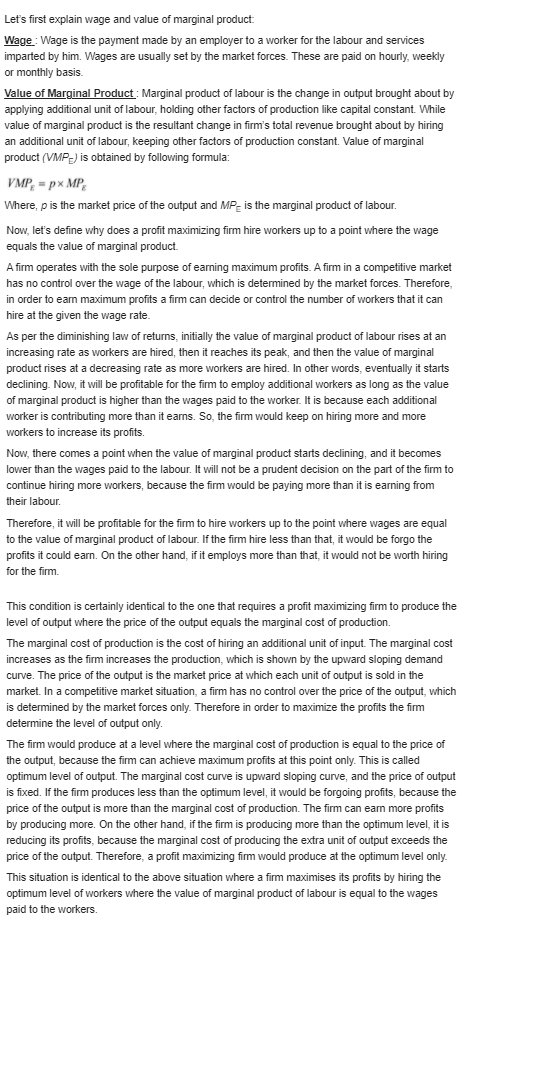# Quiz 3: Labor Demand

Given, Output to be produced (Q)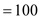units Price of capital (k)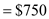per machine, per week Wage rate (w)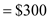per worker So, the slope of the isocost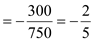Marginal rate of technical substitution between labour and capital is the slope of the isoquant. =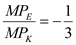Now, the inputs (labor and capital) are perfect substitutes of each other. Therefore, the isoquant curve is linear, which implies that the firm can use only labour or only capital to produce 100 units of input, depending upon which proves least costly for the firm. Let's solve this problem with the help of the below figure: Figure-1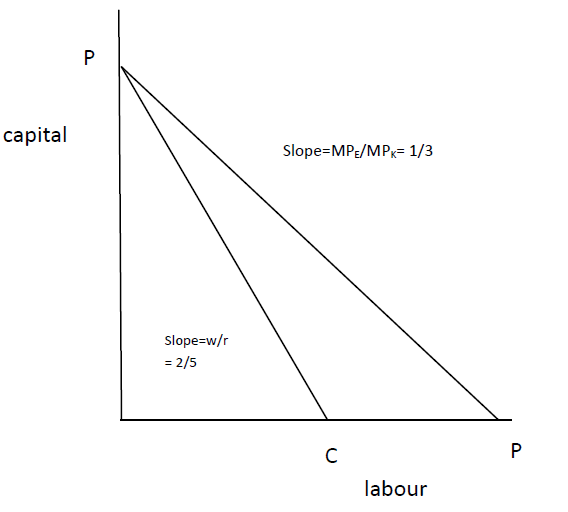In the above figure, labour is shown on the x axis, and capital on the y axis. The Isoquant line is PP, and the slope of the isoquant line is 1/3. The Isocost line is PC, and its slope is 2/5. Now the slope of the isocost line is steeper than that of the isoquant (2/5 1/3), therefore, it would cost minimum to the firm if it hires only capital at point P on y axis to produce 100 units of output. Now, let's see what firm the does when the wage rate (w) is \$225 , while others things remains same. So, the slope of the isocost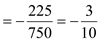Slope of the isoquant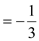Figure-2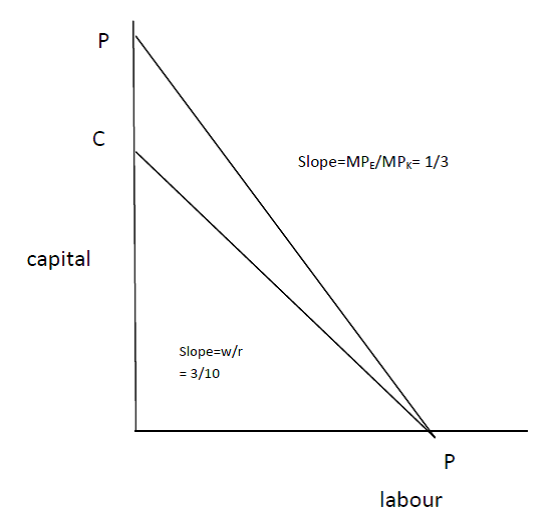In the above figure, the Isoquant line is PP , and the Isocost line is CP. The slope of the isoquant is more than isocost line (1/3) (3/10). Therefore, it would cost minimum to the firm if it hires only labour at point C on x axis to produce 100 units of output. Let's find out the elasticity of labour demand as the wage falls from \$300 to \$225. Elasticity of labour is calculated by dividing the percentage change in labour with percentage change in wages: =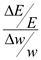Now, as the wage rate falls from \$300 to \$225, the demand for labour will move to positive number from zero. Thus, =Therefore, as the wage falls from \$300 to \$225, the elasticity of labor demand becomes infinity.